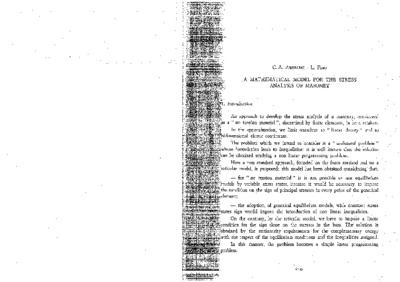# A mathematical model for the stress analysis of masonry

Anselmi, C. A. and Fino, L. (1981) A mathematical model for the stress analysis of masonry. In: Nessun futuro senza passato. 6th ICOMOS General Assembly and International Symposium. Atti. ICOMOS, Roma, pp. 519-528. [Book Section]Preview
PDF
ro31.pdf

## Abstract (in English)

An approach to stress analysis of masonry, considered as a "no tension material" is presented in this communication. The model used is a reticular model which permits the solution of problems by studying the state of equilibrium of complementary energy in conjunction with a mathematical system of inequalities. The problem we have to solve is a classical problem of quadratic programming which becomes one of linear complementarity according to the Kuln and Trucker theorem: it may therefore be solved by the "simple method"

Item Type: Book Section AuthorsEmailAnselmi, C. A.UNSPECIFIEDFino, L.UNSPECIFIED English masonry; stress analysis; mathematical model C.ARCHITECTURE > 06. Structures of buildingsF.SCIENTIFIC TECHNIQUES AND METHODOLOGIES OF CONSERVATION > 52. Structural surveys Scientific Symposium (ICOMOS General Assemblies) 1981, 6th Jose Garcia 04 Feb 2011 12:08 04 Feb 2011 12:08 https://openarchive.icomos.org/id/eprint/845View Item